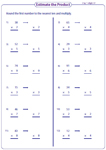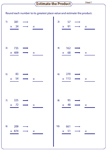1. Worksheets>
2. Math>
3. Number Sense>
4. Estimation>
5. Product / Quotient

# Estimate the Product and Quotient Worksheets

Estimating product and quotient worksheets contain exclusive worksheets on rounding numbers to the nearest ten and hundred, rounding the leading digits to estimate the product, MCQs to choose the best estimate, comparing quotients and many more.

Round to the nearest ten and estimate: Level I

Round the first factor to the nearest ten and multiply to estimate the product.2-digit by 1-digit:

3-digit by 1-digit:

Round to the nearest ten and estimate: Level II

Nine exclusive estimation worksheets have problems to round each factor to the nearest ten and multiply to estimate the product.2-digit by 2-digit:

3-digit by 2-digit:

Estimate the product - nearest hundred: Level I

Estimate the product by rounding the first factor to the nearest hundred. Six worksheets are given here for practice.3-digit by 1-digit:

3-digit by 2-digit:

Estimate the product - nearest hundred: Level II

Round each factor to the nearest hundred and multiply to estimate the product. 3-digit and 4-digit factors are used.3-digit by 3-digit:

4-digit by 3-digit:

Round the leading digit and estimate

Round the numbers to the greatest place value and estimate the product. Children can practice these mixed-digit problems.Estimate the quotients: MCQ

Each worksheet has eight multiple choice questions estimating quotients without actual division.Compare the quotients:

Write correct symbols to make the statement true (< or >). Use estimation technique to compare the quotient and a whole number in section A. Estimate and compare between the quotients in section B.Level 1:

Level 2:

Stock up your library with the complete set of worksheets on estimating products and quotients.

Related Worksheets